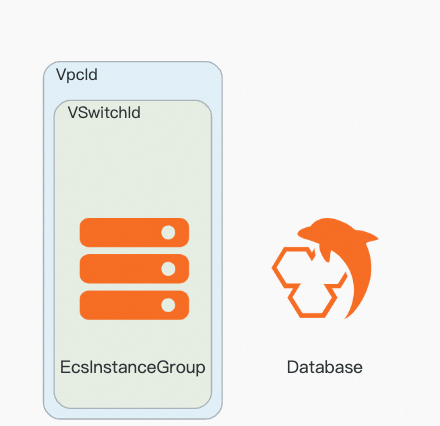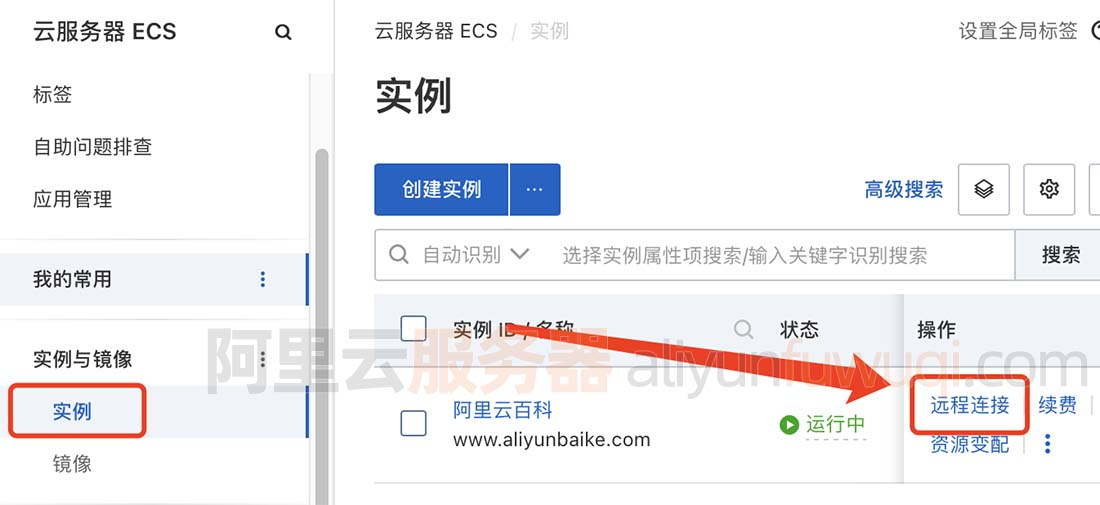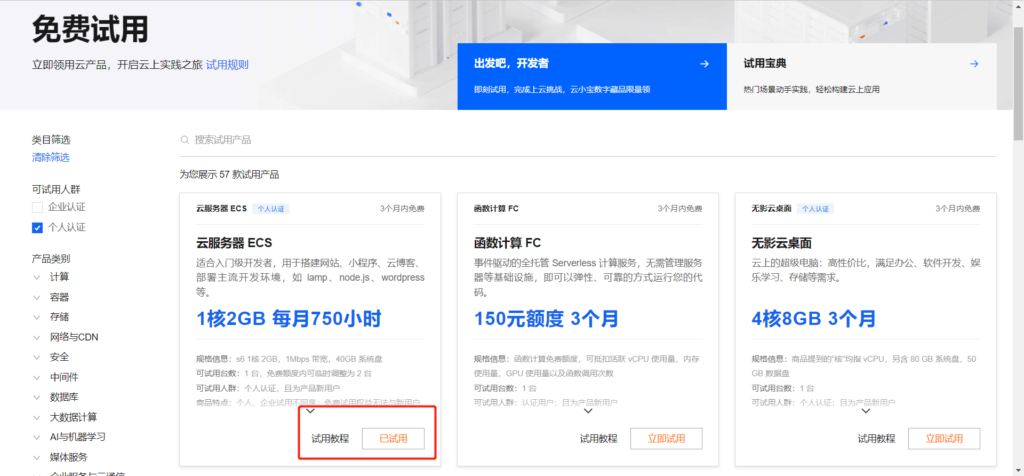+关注继续查看

WordPress常用两种钩子，过滤钩子和动作钩子。过滤钩子相关函数及源码分析在上篇文章中完成，本篇主要分析动作钩子源码。

## 动作钩子使用步骤：

2、创建动作函数：它可以有传入参数也可以无传入参数，其他与创建普通函数没有任何区别，函数的作用为完成某项动作；

4、执行动作钩子：使用do_action()可以依次执行挂载在指定钩子上的所有函数以完成指定任务；

## 动作钩子函数详解：

1、add_action($tag,$function_to_add,$priority = 10,$accepted_args = 1)

add_action()作用：该函数用于给指定的动作钩子$tag添加指定的挂载函数$function_to_add，同时它可以确定挂载函数执行优先级及其可接收参数个数；

$tag为钩子名；$function_to_add为挂载函数名；

 function add_action($tag,$function_to_add, $priority = 10,$accepted_args = 1) { return add_filter($tag,$function_to_add, $priority,$accepted_args); }   

2、do_action($tag,$arg = '')

do_action()作用：该函数调用挂载在过滤钩子$tag上的所有函数以完全特定的任务； do_action()参数说明：$tag为钩子名；

$arg为动作钩子上挂载函数的传入参数，默认为空； do_action()源码分析： function do_action($tag, $arg = '') { global$wp_filter, $wp_actions,$merged_filters, $wp_current_filter; if ( ! isset($wp_actions) )
$wp_actions = array(); # 如果$wp_actions变量未设置过，则将其定义为数组；
if ( ! isset($wp_actions[$tag]) )
$wp_actions[$tag] = 1;
else
++$wp_actions[$tag];
# 如果$wp_actions[$tag]未设置则将其赋值为1，否则将其值加1；
if ( isset($wp_filter['all']) ) {$wp_current_filter[] = $tag;$all_args = func_get_args();
_wp_call_all_hook($all_args); } # 跟apply_filters()中的all钩子处理方式完全一样！_wp_call_all_hook()源码分析见上篇文章过滤钩子源码解析； if ( !isset($wp_filter[$tag]) ) { if ( isset($wp_filter['all']) )
array_pop($wp_current_filter); return; } # 当前钩子不存在，则直接返回，不再执行以后代码； if ( !isset($wp_filter['all']) )
$wp_current_filter[] =$tag;
# 将当前钩子设置为$tag；$args = array();
if ( is_array($arg) && 1 == count($arg) && isset($arg) && is_object($arg) )
$args[] =&$arg;
else
$args[] =$arg;
# do_action()若有传入参数，且为一个数组，该数组仅此一个元素，该元素有值则将$args值设置为引用$arg，否则直接赋值；
for ( $a = 2;$a < func_num_args(); $a++ )$args[] = func_get_arg($a); # 通过for循环，若do_action()有不只一个传入参数，将这些值赋给数组$args；
if ( !isset( $merged_filters[$tag ] ) ) {
ksort($wp_filter[$tag]);
$merged_filters[$tag ] = true;
}
# 跟apply_filter()函数排序代码完全一样！详解见上文；
reset( $wp_filter[$tag ] );
do {
foreach ( (array) current($wp_filter[$tag]) as $the_ ) if ( !is_null($the_['function']) )
call_user_func_array($the_['function'], array_slice($args, 0, (int) $the_['accepted_args'])); } while ( next($wp_filter[$tag]) !== false ); array_pop($wp_current_filter);
}
# 除了少了一行return \$value其他跟apply_filters()完全一样！65 0wordpress源码解析-目录结构-文件调用关系（转）
2222 0wordpress源码解析-数据库表结构（转）
1396 01995 0WordPress是一种流行的开源内容管理系统（CMS），用于创建和管理网站及博客，是目前最多人和最容易使用的网站建立工具。WordPress不仅易于使用，而且强大和灵活，它拥有丰富的主题和插件库，可以轻松地自定义网站外观和功能。它也有一个庞大的社区，提供了广泛的支持和资源。有许多网站和博客使用WordPress来管理其内容和功能。阿里云计算巢推出了WordPress社区版一方服务，不需要再进行复
36 032 031 046 085 0183 0